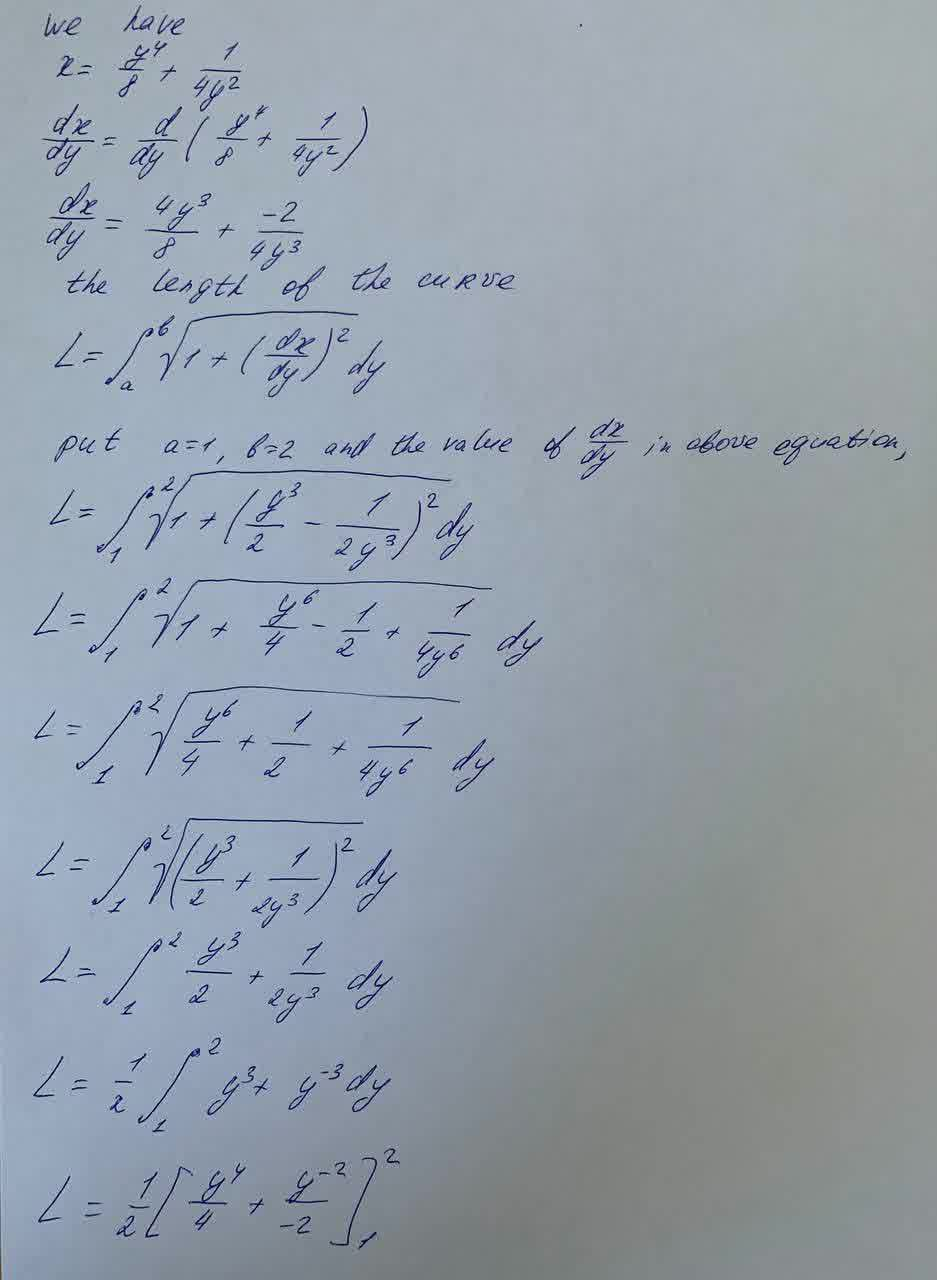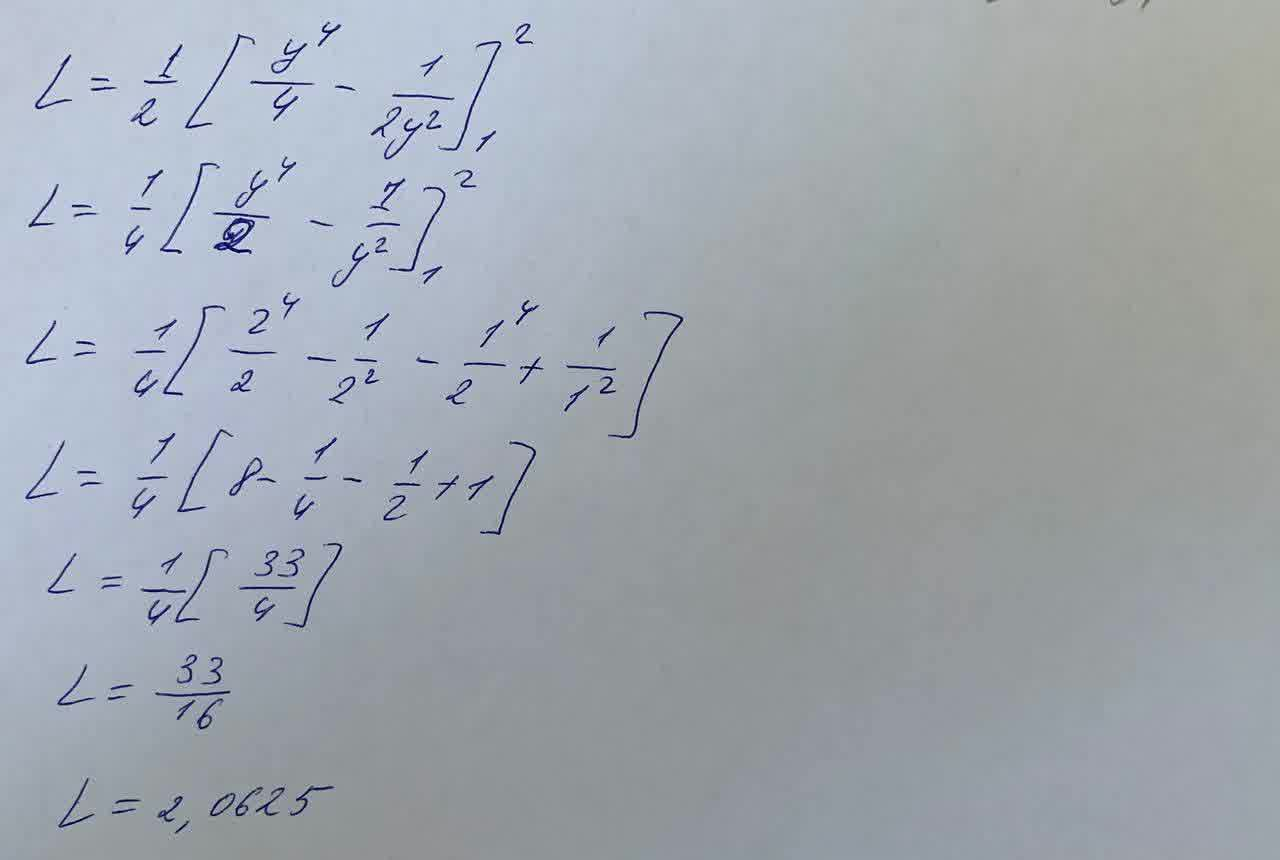Question# Find the exact length of the curve. x = \frac{y^4}{8} + \frac{1}{4y^2} , 1 \leq y \leq 2

Integrals
ANSWEREDFind the exact length of the curve. $$\displaystyle{x}={\frac{{{y}^{{4}}}}{{{8}}}}+{\frac{{{1}}}{{{4}{y}^{{2}}}}},{1}\leq{y}\leq{2}$$